# Hybrids that push the limit

Discussion in 'Hybriding' started by HACKr, Apr 4, 2006.

1. ### HYBRIDLVR

Messages:
1,443
You can use the Min/Max code to raise the suspension to 256 Max, It's also how the Projects cars are set up, They use settings beyond the games limits!

Messages:
4,783
Location: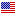Thanks!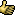Yeah I forgot about the max code with zero. I tried it on the spring settings and in the program it only went to -1. I guess you can't do it on some things.

3. ### HYBRIDLVR

Messages:
1,443
Which GT game are you talking about? GT 1 and 2 there's a actual code to let you change from 0 to 256 for minimum to maximum settings, GT3 let you use "0" for some codes for maximum settinigs like Downforce and a few others, It doesn't work for all Max codes in GT3, Do you use MK's or a Device/GS or AR?

Messages:
4,783
Location:It's GT3 and I use MK's program. I was going to mention it but must have forgot. I got all my info from famine's site, including MK's program, and when I saw the second Genome progect, I was inspired to try the civic.

5. ### opendriver

Messages:
3,704
You should do the mini, I put a 6000hp TVR speed 12 engine in it and it FLYS!!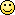6. ### graveslids

Messages:
74
A member known as Werner Winkels used to have some seriously fast hybrids posted up. I haven't been in this section of the board in a long time, so he could be active. Anyhow, the majority of the cars he posted were stupid fast and handled exceedingly well.

7. ### fideles1986

Messages:
531
Location: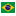New here, but what exactly is a Hybrid?

thanx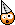Messages:
69,810
Location: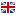A car made up of a collection of parts from other cars - not achievable through normal means in the game.

Messages:
4,783
Location:Here's an ini for a ZZII that bounces like the civic.[GT3CarData]
Region=US
BaseType= zz2_00
BaseName= ZZII(J)
0=1504192463
4=-497624
8=-648
12=-3
16=-2
20=-1
24=158906213
28=-1609951638
32=-12514
36=-1
40=158979882
44=-1609951638
48=158914215
52=-1609951638
56=-1963651629
60=-609062626
64=158971436
68=537532010
72=158741284
76=537532010
80=158815461
84=537532010
88=158324324
92=-1609952158
96=-1314588646
100=1217339591
104=-2619186
108=-1
112=-1
116=-1
120=158815080
124=537532010
128=-1
132=-1
136=-1
140=-1
144=-1
148=-1
152=-1
156=-1
160=-1
164=-1
168=158798567
172=537532010
176=-1
180=-1
184=158947112
188=-1609951638
192=-1
196=-1
200=158790820
204=537532010
208=-1
212=-1
216=-12514
220=-1
224=-12514
228=-1
232=-1
236=-1
240=-2921
244=-2846
248=-2069
252=-1705
256=-1349
260=-1114
264=-961
268=-1
272=-3230
276=-22
280=-1
284=-13
288=-13
292=-101
296=-126
300=-9
304=-56
308=-96
312=-16
316=-81
320=-61
324=-21
328=0
332=-116
336=-257
340=-129
344=-129
348=0
352=0
356=-76
360=-76
364=-999999999
368=-999999999
372=-999999999
376=-999999999
380=-999999999
384=-999999999
388=-999999999
392=-999999999
396=-5
400=-5
404=-6
408=-11
412=-6
416=-41
420=-6
424=-21
428=-5500
432=-11
436=-1
440=-1
444=-2
448=-2
452=-2
456=-2
460=-1
464=-79734154
468=27111035
472=-2744308
476=-66250
480=-1208032491
484=936574911
488=-1061997774
492=-1
496=-1
500=-1
504=-1
508=-2
512=-3

And here's a Spoon Civic that is (basically) the same as Famine's version only a different model.

[GT3CarData]
Region=US
BaseType= spoon_civic_00
BaseName= Spoon Civic Type-R(J)
0=-998626344
4=-1606925607
8=-815
12=-2
16=-1
20=-1
24=-1963651500
28=1555198238
32=-14191
36=-1
40=-1963651212
44=565342494
48=-1963651469
52=-1632472802
56=-1963651629
60=-609062626
64=-1963651245
68=590508318
72=-1963652144
76=456290590
80=-1963651855
84=-600674018
88=-1963651693
92=1538421022
96=430241831
100=1217339592
104=-2619186
108=-1
112=-1
116=-1
120=-1963651856
124=1597141278
128=-1
132=-1
136=-1
140=-1
144=-1
148=-1
152=-1
156=-1
160=-1
164=-1
168=-3405666
172=-1
176=-1963651822
180=1513255198
184=-1963651340
188=531788062
192=-1
196=-1
200=-1963651984
204=-1691193058
208=-1
212=-1
216=-12514
220=-1
224=-12514
228=-1
232=-1
236=-1
240=-3001
244=-3231
248=-2106
252=-1459
256=-1108
260=-849
264=-64538
268=-64538
272=-4401
276=-1
280=-1
284=-2
288=-2
292=-20
296=-27
300=-1
304=-1
308=-1
312=-1
316=-1
320=-1
324=-11
328=0
332=-116
336=-257
340=-129
344=-129
348=0
352=0
356=-76
360=-76
364=-999999999
368=-999999999
372=-999999999
376=-999999999
380=-999999999
384=-999999999
388=-999999999
392=-999999999
396=-2
400=-2
404=-8
408=-1
412=-31
416=-1
420=-16
424=-1
428=-4401
432=-12
436=-1
440=-1
444=-2
448=-2
452=-2
456=-2
460=-1
464=-1149904198
468=-22106433
472=-2646029
476=-1
480=929924095
484=929924095
488=-1
492=-1
496=-1
500=-1
504=-1
508=-2
512=-58

10. ### opendriver

Messages:
3,704
Ill post some more vids of the hybrid garage that WW posted in anoth thred
Some crazy stuff there, look for an update soon

Messages:
4,783
Location:Bouncy RX8

[GT3CarData]
Region=US
BaseType= rx8_01_ext
BaseName= Mazda RX-8
0=-469393710
4=-2020703620
8=-976
12=-2
16=-1
20=-1
24=182743554
28=-1600901770
32=-14191
36=-1
40=158988074
44=-1608901062
48=719614466
52=-1600901270
56=-1963651629
60=-609062626
64=-1963651245
68=590508318
72=-1963652144
76=456290590
80=-1963651855
84=-600674018
88=-1963651693
92=1538421022
96=430241831
100=1217339592
104=-2619186
108=-1
112=-1
116=-1
120=853832194
124=-1600907466
128=-1
132=-1
136=-1
140=-1
144=-1
148=-1
152=-1
156=-1
160=-1
164=-1
168=-3405666
172=-1
176=-1963651822
180=1513255198
184=-1963651340
188=531788062
192=-1
196=-1
200=-1963651984
204=-1691193058
208=-1
212=-1
216=-12514
220=-1
224=-12514
228=-1
232=-1
236=-1
240=-3001
244=-3231
248=-2106
252=-1459
256=-1108
260=-849
264=-64538
268=-64538
272=-4401
276=-1
280=-1
284=-2
288=-2
292=-30
296=-48
300=-1
304=-1
308=-1
312=-1
316=-1
320=-1
324=-11
328=0
332=-116
336=-257
340=-129
344=-129
348=0
352=0
356=-76
360=-76
364=-999999999
368=-999999999
372=-999999999
376=-999999999
380=-999999999
384=-999999999
388=-999999999
392=-999999999
396=-2
400=-2
404=-8
408=-1
412=-31
416=-1
420=-16
424=-1
428=-4401
432=-12
436=-1
440=-1
444=-2
448=-2
452=-2
456=-2
460=-1
464=-1149904198
468=-22106433
472=-2646029
476=-1
480=929924095
484=929924095
488=-1
492=-1
496=-1
500=-1
504=-1
508=-2
512=-5

Bouncy Camaro

[GT3CarData]
Region=US
BaseType= camaro_z28_coupe_97
BaseName= Camaro Z28
0=1967650451
4=-1354072955
8=-138
12=-8
16=-1
20=-1
24=-1963651500
28=1555198238
32=-14191
36=-1
40=-1834173455
44=-1625787766
48=-1680568235
52=-1780700854
56=-1963651629
60=-609062626
64=-1963651245
68=590508318
72=-1963652144
76=456290590
80=-1963651855
84=-600674018
88=-1963651693
92=1538421022
96=430241831
100=1217339592
104=-2619186
108=-1
112=-1
116=-1
120=2125249521
124=-1625792916
128=-1
132=-1
136=-1
140=-1
144=-1
148=-1
152=-1
156=-1
160=-1
164=-1
168=-3405666
172=-1
176=-1963651822
180=1513255198
184=-1963651340
188=531788062
192=-1
196=-1
200=-1963651984
204=-1691193058
208=-1
212=-1
216=-12514
220=-1
224=-12514
228=-1
232=-1
236=-1
240=-3001
244=-3231
248=-2106
252=-1459
256=-1108
260=-849
264=-64538
268=-64538
272=-4401
276=-1
280=-1
284=-2
288=-2
292=-25
296=-36
300=-1
304=-1
308=-1
312=-1
316=-1
320=-1
324=-11
328=0
332=-116
336=-257
340=-129
344=-129
348=0
352=0
356=-76
360=-76
364=-999999999
368=-999999999
372=-999999999
376=-999999999
380=-999999999
384=-999999999
388=-999999999
392=-999999999
396=-2
400=-2
404=-8
408=-1
412=-31
416=-1
420=-16
424=-1
428=-4401
432=-12
436=-1
440=-1
444=-2
448=-2
452=-2
456=-2
460=-1
464=-1149904198
468=-22106433
472=-2646029
476=-1
480=929924095
484=929924095
488=-1
492=-1
496=-1
500=-1
504=-1
508=-2
512=-4

One of my freakiest cars! Try it out!

[GT3CarData]
Region=US
BaseType= lotus_99t
BaseName= F687/S
0=-1005477555
4=-1826608048
8=-1377
12=-1
16=-2
20=-1
24=1368839399
24=-1963651500
28=185867077
32=-14191
36=-1
40=1245107431
44=185867113
48=970380519
52=185867081
56=-1963651629
60=-609062626
64=-1963651245
68=590508318
72=-1963652144
76=456290590
80=-1963651855
84=-600674018
88=-1963651693
92=1538421022
96=430241831
100=1217339592
104=-2619186
108=-1
112=-1
116=-1
120=-773401369
124=185867032
128=-1
132=-1
136=-1
140=-1
144=-1
148=-1
152=-1
156=-1
160=-1
164=-1
168=-3405666
172=-1
176=-1963651822
180=1513255198
184=-1963651340
188=531788062
192=-1
196=-1
200=-1963651984
204=-1691193058
208=-1
212=-1
216=-12514
220=-1
224=-12514
228=-1
232=-1
236=-1
240=-3001
244=-3231
248=-2106
252=-1459
256=-1108
260=-849
264=-64538
268=-64538
272=-4401
276=-1
280=-1
284=-2
288=-2
292=-71
296=-91
300=-1
304=-1
308=-1
312=-1
316=-1
320=-1
324=-11
328=0
332=-116
336=-257
340=-129
344=-129
348=0
352=0
356=-76
360=-76
364=-999999999
368=-999999999
372=-999999999
376=-999999999
380=-999999999
384=-999999999
388=-999999999
392=-999999999
396=-2
400=-2
404=-8
408=-1
412=-31
416=-1
420=-16
424=-1
428=-4401
432=-12
436=-1
440=-1
444=-2
448=-2
452=-2
456=-2
460=-1
464=-1149904198
468=-22237505
472=-2646029
476=-1
480=929924095
484=929924095
488=-1
492=-1
496=-1
500=-1
504=-1
508=-2
512=-6

12. ### ajc_176

Messages:
9
hey where did you get your Ps1 roms? i cant find any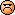13. ### Thiele

Messages:
3,210
you have to put the CD in the computer, and use a program like ISO buster to extract an image from it, you can get it off the internet in some places, but on most rom sites you can't get them because of their file size and it would take years to download [up to 700mb] so you have to have enough space on your HD.

i do know one site that has psx ISOs,

is there anyway to view those videos in km/hr, i want to see how fast that gt40 really goes

Messages:
4,783
Location:A few more bouncy cars.
Look at base name to see what car it is as they all bounce the same. The only difference is that the faster cars bounce a bit differently than the slow ones.

[GT3CarData]
Region=US
0=-1541658801
4=-1707073738
8=-1131
12=-1
16=-1
20=-1
24=164810230
28=1405135111
32=-14191
36=-1
40=164810518
44=415279367
48=164810261
52=-1782535929
56=-1963651629
60=-609062626
64=164810485
68=440445191
72=-774565215
76=-837007006
80=164809875
84=-750737145
88=-1963651693
92=1538421022
96=430241831
100=1217339592
104=-2619186
108=-1
112=-1
116=-1
120=164809874
124=1447078151
128=-1
132=-1
136=-1
140=-1
144=-1
148=-1
152=-1
156=-1
160=-1
164=-1
168=-3405666
172=-1
176=-1963651822
180=1513255198
184=-1963651340
188=531788062
192=-1
196=-1
200=-1963651984
204=-1691193058
208=-1
212=-1
216=-12514
220=-1
224=-12514
228=-1
232=-1
236=-1
240=-2954
244=-3248
248=-2073
252=-1467
256=-1092
260=-856
264=-706
268=-64538
272=-3770
276=-1
280=-1
284=-2
288=-2
292=-75
296=-72
300=-1
304=-1
308=-1
312=-1
316=-1
320=-1
324=-11
328=0
332=-246
336=-77
340=-129
344=-129
348=0
352=0
356=-76
360=-76
364=-999999999
368=-999999999
372=-999999999
376=-999999999
380=-999999999
384=-999999999
388=-999999999
392=-999999999
396=-2
400=-2
404=-1
408=-11
412=-1
416=-41
420=-1
424=-21
428=-3770
432=-12
436=-1
440=-1
444=-2
448=-2
452=-2
456=-2
460=-1
464=-1149904198
468=-22106433
472=-2646029
476=-1
480=929924095
484=929924095
488=-1
492=-1
496=-1
500=-1
504=-1
508=-2
512=-3

[GT3CarData]
Region=US
BaseType= lancer_evo6_tommi_gsr_00
BaseName= LANCER Evolution VI GSR T.M.E. (J)
0=-2077012196
4=1648185129
8=-1081
12=-1
16=-1
20=-1
24=-747818366
28=1288331532
32=-14191
36=-1
40=-747799949
44=-1932893940
48=-747816366
52=-859152116
56=-1963651629
60=-609062626
64=-747802061
68=-322281204
72=-774565215
76=-837007006
80=-747841054
84=751460620
88=-1963651693
92=1538421022
96=430241831
100=1217339592
104=-2619186
108=-1
112=-1
116=-1
120=-747841150
124=-322281204
128=-1
132=-1
136=-1
140=-1
144=-1
148=-1
152=-1
156=-1
160=-1
164=-1
168=-3405666
172=-1
176=-1963651822
180=1513255198
184=-1963651340
188=531788062
192=-1
196=-1
200=-1963651984
204=-1691193058
208=-1
212=-1
216=-12514
220=-1
224=-12514
228=-1
232=-1
236=-1
240=-3417
244=-2786
248=-1951
252=-1408
256=-1032
260=-762
264=-64538
268=-64538
272=-4530
276=-1
280=-1
284=-2
288=-2
292=-20
296=-27
300=-1
304=-1
308=-1
312=-1
316=-1
320=-1
324=-11
328=0
332=-246
336=-77
340=-129
344=-129
348=0
352=0
356=-76
360=-76
364=-999999999
368=-999999999
372=-999999999
376=-999999999
380=-999999999
384=-999999999
388=-999999999
392=-999999999
396=-2
400=-2
404=-4
408=-31
412=-19
416=-1
420=-9
424=-1
428=-4530
432=-12
436=-1
440=-1
444=-2
448=-2
452=-2
456=-2
460=-1
464=-1149904198
468=-22106433
472=-2646029
476=-1
480=929924095
484=929924095
488=-1
492=-1
496=-1
500=-1
504=-1
508=-2
512=-4

[GT3CarData]
Region=US
BaseType= elise_190_00
BaseName= Elise 190
0=758869147
4=204767860
8=-913
12=-1
16=-1
20=-1
24=-618228951
28=1102722298
32=-14191
36=-1
40=-618228951
44=1178159354
48=-618228951
52=1110916346
56=-1963651629
60=-609062626
64=-618228951
68=1169510138
72=-774565215
76=-837007006
80=-618228951
84=1009791738
88=-618228951
92=1052127482
96=430241831
100=1217339592
104=-2619186
108=-1
112=-1
116=-1
120=-618228951
124=1009401594
128=-1
132=-1
136=-1
140=-1
144=-1
148=-1
152=-1
156=-1
160=-1
164=-1
168=-3405666
172=-1
176=-1963651822
180=1513255198
184=-1963651340
188=531788062
192=-1
196=-1
200=-1963651984
204=-1691193058
208=-1
212=-1
216=-12514
220=-1
224=-12514
228=-1
232=-1
236=-1
240=-3001
244=-3168
248=-1843
252=-1309
256=-1034
260=-766
264=-64538
268=-64538
272=-3939
276=-1
280=-1
284=-2
288=-2
292=-20
296=-27
300=-1
304=-1
308=-1
312=-1
316=-1
320=-1
324=-11
328=0
332=-246
336=-77
340=-129
344=-129
348=0
352=0
356=-76
360=-76
364=-999999999
368=-999999999
372=-999999999
376=-999999999
380=-999999999
384=-999999999
388=-999999999
392=-999999999
396=-2
400=-2
404=-1
408=-8
412=-1
416=-31
420=-1
424=-16
428=-3939
432=-12
436=-1
440=-1
444=-2
448=-2
452=-2
456=-2
460=-1
464=-1149904198
468=-22106433
472=-2646029
476=-1
480=929924095
484=929924095
488=-1
492=-1
496=-1
500=-1
504=-1
508=-2
512=-5

[GT3CarData]
Region=US
BaseType= _787b_91
BaseName= 787B
0=-2086132916
4=-817814980
8=-1131
12=-1
16=-2
20=-1
24=-358270929
28=1217339592
32=-14191
36=-1
40=848721967
44=1217339593
48=-227166161
52=1217339592
56=-1963651629
60=-609062626
64=710334511
68=1217339593
72=-774565215
76=-837007006
80=-1845159889
84=1217339592
88=-1302005714
92=1217339592
96=430241831
100=1217339592
104=-2619186
108=-1
112=-1
116=-1
120=-1851402193
124=1217339592
128=-1
132=-1
136=-1
140=-1
144=-1
148=-1
152=-1
156=-1
160=-1
164=-1
168=-1853482961
172=1217339592
176=-1963651822
180=1513255198
184=-1963651340
188=531788062
192=-1
196=-1
200=-1963651984
204=-1691193058
208=-1
212=-1
216=-12514
220=-1
224=-12514
228=-1
232=-1
236=-1
240=-3345
244=-3691
248=-2309
252=-1602
256=-1181
260=-924
264=-64538
268=-64538
272=-3801
276=-37
280=-1
284=-2
288=-2
292=-75
296=-92
300=-4
304=-56
308=-121
312=-13
316=-76
320=-76
324=-11
328=0
332=-246
336=-77
340=-129
344=-129
348=0
352=0
356=-76
360=-76
364=-999999999
368=-999999999
372=-999999999
376=-999999999
380=-999999999
384=-999999999
388=-999999999
392=-999999999
396=-2
400=-2
404=-1
408=-11
412=-1
416=-41
420=-1
424=-21
428=-3801
432=-12
436=-1
440=-1
444=-2
448=-2
452=-2
456=-2
460=-1
464=-1154098502
468=-55791941
472=-2646045
476=-1
480=929924095
484=929924095
488=-1
492=-1
496=-1
500=-1
504=-1
508=-2
512=-6

[GT3CarData]
Region=US
BaseType= griffith_500_00_ext
BaseName= Griffith 500
0=-1531407145
4=1446294753
8=-1490
12=-1
16=-1
20=-1
24=-1880250964
28=758103912
32=-14191
36=-1
40=-1209162324
44=758106214
48=-1611815508
52=758104162
56=1100305258
60=-693417118
64=-1007835732
68=758105950
72=-774565215
76=-837007006
80=-1947359828
84=758101076
88=-2014468692
92=758102368
96=430241831
100=1217339592
104=-2619186
108=-1
112=-1
116=-1
120=-1544706644
124=758101064
128=-1
132=-1
136=-1
140=-1
144=-1
148=-1
152=-1
156=-1
160=-1
164=-1
168=-3405666
172=-1
176=-1963651822
180=1513255198
184=-1963651340
188=531788062
192=-1
196=-1
200=-1963651984
204=-1691193058
208=-1
212=-1
216=-12514
220=-1
224=-12514
228=-1
232=-1
236=-1
240=-2761
244=-2951
248=-1941
252=-1341
256=-1001
260=-731
264=-64538
268=-64538
272=-3311
276=-1
280=-1
284=-2
288=-2
292=-30
296=-48
300=-1
304=-1
308=-1
312=-1
316=-1
320=-1
324=-11
328=0
332=-246
336=-77
340=-129
344=-129
348=0
352=0
356=-76
360=-76
364=-999999999
368=-999999999
372=-999999999
376=-999999999
380=-999999999
384=-999999999
388=-999999999
392=-999999999
396=-2
400=-2
404=-1
408=-6
412=-1
416=-21
420=-1
424=-11
428=-3311
432=-12
436=-1
440=-1
444=-2
448=-2
452=-2
456=-2
460=-1
464=-1149904198
468=-22106433
472=-2646029
476=-1
480=929924095
484=929924095
488=-1
492=-1
496=-1
500=-1
504=-1
508=-2
512=-7

Edit: Any cars you wan't me to do?

15. ### opendriver

Messages:
3,704
Project hellica:

16. ### samj_13

Messages:
1,069
OD19a, that is one insane Toyota.

17. ### opendriver

Messages:
3,704
I got the ideaa fromm mmonster garage when they put that jet engine in a new celica. It has a little less power than the jet engine, but it still gos really fast

18. ### Thiele

Messages:
3,210
ca you view these videos in km/hr? so i can see how fast they're really going?

Messages:
69,810
Location:They're really going that fast.

But in a different measurement system - that of km/h - the Celica reaches 586km/h.

Messages:
4,783
Location:Hey opendriver, would you mind trying out my cars and posting a video of one?

...If it's not to much trouble.

Tell me what you think!

21. ### opendriver

Messages:
3,704
Give me your game save file with some hybrids on it and ill do some sort of "testing" with them and do the best one that I like

any format is ok

Messages:
4,783
Location:Here you go!
The first ones are rally cars I made (you can tell because they don't have a lot of camber), but if you want to try them anyway you are welcome to.The rest are bouncy cars. sorry there are so many though.

File size:
8.9 KB
Views:
5
23. ### TMM

Messages:
1,203
Location: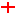Called Mildbirds, been done in GT2 for a while. After all insane power gets boring...

24. ### HYBRIDLVR

Messages:
1,443
MildBrids are much more fun!

25. ### AngelCreator

Messages:
2,276
That is true.

26. ### opendriver

Messages:
3,704
Try putting a RX8 engine into a indy car. You have a 280-300hp car that can smoke the JGTC500 cars by about 20 seconds on a small track. It is a receation of a mazda spec racer that is raced over at infineon

27. ### opendriver

Messages:
3,704
ouhweo[tgbsobgsnpdjfbgs[gn\
thingyomfg

28. ### opendriver

Messages:
3,704
Delete These Post Mods!29. ### opendriver

Messages:
3,704
Delete These Post Mods!

30. ### opendriver

Messages:
3,704
Delete These Post Mods!